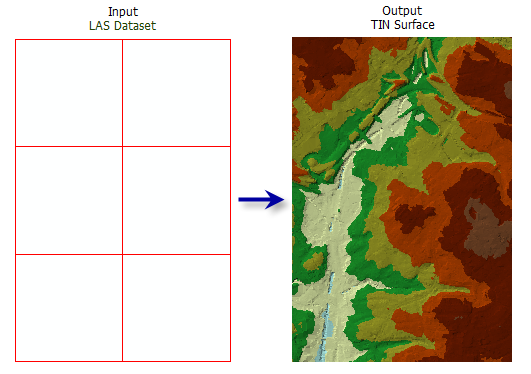# LAS Dataset To TIN (3D Analyst)

## Summary

Exports a triangulated irregular network (TIN) from a LAS dataset.

## Illustration## Usage

• You can have the LAS dataset layer limit the LAS points that are displayed and processed by selecting any combination of classification codes, classification flags, and return values in the layer's filter settings. The filters can be defined through the Layer Properties dialog box or the Make LAS Dataset Layer tool.

• The LAS dataset layer can also be used to control the enforcement of surface constraint features that may be referenced by the LAS dataset. The constraints are enforced when displaying or processing the LAS dataset as a triangulated surface.

• While the total number of points that a TIN can support can exceed 15 million points, it is recommended that you limit TIN datasets to no more than 5 million points to ensure a responsive performance when displaying and analyzing the data. The TIN node count can be reduced using point thinning methods and controlling the output processing extent.

##### Note:

Consider using a Window Size thinning type (thinning_type="WINDOW_SIZE" in Python) when you need more predictable control of how LAS points are thinned in the generation of the output TIN.

## Syntax

`arcpy.3d.LasDatasetToTin(in_las_dataset, out_tin, {thinning_type}, {thinning_method}, {thinning_value}, {max_nodes}, {z_factor}, {clip_to_extent})`
 Parameter Explanation Data Type in_las_dataset The LAS dataset to process. LAS Dataset Layer out_tin The TIN dataset that will be generated. TIN thinning_type(Optional) Specifies the technique to be used to select a subset of LAS data points that will be exported to TIN. NONE —No thinning is applied. This is the default.RANDOM —LAS data points are randomly selected based on the corresponding thinning_method selection and thinning_value entry.WINDOW_SIZE —The LAS dataset is divided into square tiles defined by the thinning_value, and LAS points are selected using the thinning_method. String thinning_method(Optional) Specifies the technique to be used to reduce the LAS data points, which impacts the interpretation of Thinning Value. The available options depend on the selected Thinning Type. PERCENT —Thinning value will reflect the percentage of LAS points that will be preserved in the outputNODE_COUNT —Thinning value will reflect the total number of nodes that are allowed in the outputMIN —Selects the LAS data point with the lowest elevation in each window size areaMAX —Selects the LAS data point with the highest elevation in each of the automatically determined window size areasCLOSEST_TO_MEAN —Selects the LAS data point with the elevation closest to the average value found in the automatically determined window size areasSpecifies the technique to be used to reduce the LAS data points, which impacts the interpretation of the thinning_value. The available options depend on the selected thinning_type.PERCENT — The thinning_value will reflect a percentage of the total points in the LAS dataset. This option is only available when thinning_type="RANDOM".NODE_COUNT —The thinning_value will reflect the total number of nodes that are allowed in the output. This option is only available when thinning_type="RANDOM".MIN —Selects the LAS point with the lowest elevation in each window size area. This option is only available when thinning_type="WINDOW_SIZE".MAX —Selects the LAS point with the highest elevation in each window size area. This option is only available when thinning_type="WINDOW_SIZE".CLOSEST_TO_MEAN —Selects the LAS point with the elevation closest to the average value of all LAS points in each window size area. This option is only available when thinning_type="WINDOW_SIZE". String thinning_value(Optional) If thinning_type="WINDOW_SIZE", this value represents the sampling area by which the LAS dataset will be divided. If thinning_type="RANDOM" and thinning_method="PERCENT", this value represents the percentage of points from the LAS dataset that will be exported to the TIN. If thinning_type="RANDOM" and thinning_method="NODE_COUNT", this value represents the total number of LAS points that can be exported to the TIN. Double max_nodes(Optional) The maximum number of nodes permitted in the output TIN. The default is 5 million. Double z_factor(Optional) The factor by which z-values will be multiplied. This is typically used to convert Z linear units to match XY linear units. The default is 1, which leaves elevation values unchanged. This parameter is disabled if the spatial reference of the input surface has a Z datum with a specified linear unit. Double clip_to_extent(Optional) Specifies whether the resulting TIN will be clipped against the analysis extent. This only has an effect if the analysis extent is a subset of the input LAS dataset. CLIP —Clips the output TIN against the analysis extent. This is the default. NO_CLIP —Does not clip the output TIN against the analysis extent. Boolean

## Code sample

LasDatasetToTin example 1 (Python window)

The following sample demonstrates the use of this tool in the Python window.

``````arcpy.env.workspace = 'C:/data'
arcpy.LasDatasetToTin_3d('se_baltimore.lasd', 'se_bmore', 'RANDOM', 15, 3.28)``````
LasDatasetToTin example 2 (stand-alone script)

The following sample demonstrates the use of this tool in a stand-alone Python script.

``````'''**********************************************************************
Name: LAS Dataset to TIN Example
Description: Create a TIN using bare earth lidar measurements. This
script is designed for use as a script tool.
**********************************************************************'''
# Import system modules
import arcpy

# Set Local Variables
lasD = arcpy.GetParameterAsText(0)
inLas = arcpy.GetParameterAsText(1) #input las files
surfCons = arcpy.GetParameterAsText(2) #input surface constraints
sr = arcpy.GetParameter(3) #spatial reference of las dataset
outTin = arcpy.GetParameterAsText(4)
thinningType = arcpy.GetParameterAsText(5)
thinningMethod = arcpy.GetParameterAsText(6)
thinningValue = arcpy.GetParameter(7)
zFactor = arcpy.GetParameter(8)

# Execute CreateLasDataset
arcpy.management.CreateLasDataset(inLas, lasD, 'RECURSION', surfCons, sr)
lasLyr = arcpy.CreateUniqueName('lasdToTin', 'in_memory')
classCode = 2
returnValue = 'LAST'
# Execute MakeLasDatasetLayer
arcpy.management.MakeLasDatasetLayer(lasD, lasLyr, classCode, returnValue)
# Define extent of the area of interest
env.extent(1426057, 606477, 1449836, 623246)
# Execute LasDatasetToTin
arcpy.ddd.LasDatasetToTin(lasLyr, outTin, thinningType,
thinningMethod, thinningValue, zFactor)``````

## Licensing information

• Basic: Requires 3D Analyst
• Standard: Requires 3D Analyst